Next: Non-Degenerate Perturbation Theory Up: Time-Independent Perturbation Theory Previous: Introduction

# Two-State System

Consider the simplest non-trivial system, in which there are only two independent eigenkets of the unperturbed Hamiltonian. These are denoted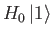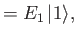(7.2)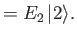(7.3)

It is assumed that these states, and their associated eigenvalues, are known. Because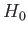is, by definition, an Hermitian operator, its two eigenkets are mutually orthogonal and form a complete set. The lengths of these eigenkets are both normalized to unity. Let us now try to solve the modified energy eigenvalue problem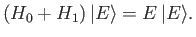(7.4)

In fact, we can solve this problem exactly. Because the eigenkets ofform a complete set, we can write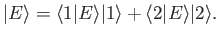(7.5)

Substituting the previous expansion into Equation (7.4), and then right-multiplying by either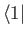or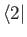, we get two coupled equations that can be written in matrix form: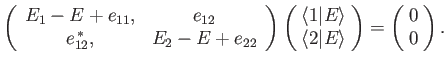(7.6)

Here,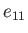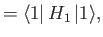(7.7)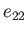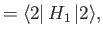(7.8)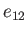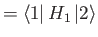(7.9)

are the so-called matrix elements of the perturbing Hamiltonian (with respect to the unperturbed eigenstates). In the special (but common) case of a perturbing Hamiltonian whose diagonal matrix elements are zero, so that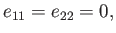(7.10)

the non-trivial solution of Equation (7.6) (obtained by setting the determinant of the matrix equal to zero ) is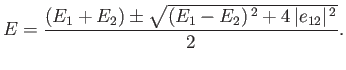(7.11)

Let us expand in the supposedly small parameter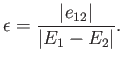(7.12)

We obtain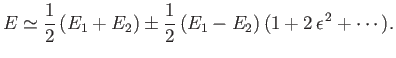(7.13)

The previous expression yields the modifications to the energy eigenvalues caused by the perturbing Hamiltonian: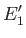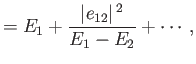(7.14)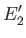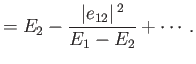(7.15)

Note that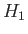causes the upper eigenvalue to increase, and the lower eigenvalue to decrease. It is easily demonstrated that the modified eigenkets take the form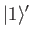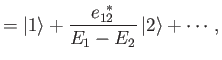(7.16)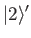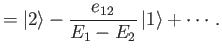(7.17)

Thus, the modified energy eigenstates consist of one of the unperturbed eigenstates with a slight admixture of the other. Actually, the series expansion on the right-hand side of Equation (7.13) only converges when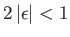. This suggests that the condition for the validity of the perturbation expansion is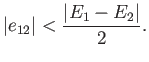(7.18)

In other words, when we say thatmust be small compared to, what we really mean is that the previous inequality needs to be satisfied.Next: Non-Degenerate Perturbation Theory Up: Time-Independent Perturbation Theory Previous: Introduction
Richard Fitzpatrick 2016-01-22